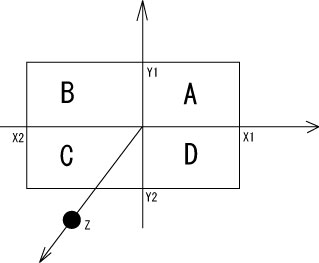Solid angle calculatorThe calculator of solid angles of areas of ABCD from the point z.

input

X1,X2,X3,X4 are lengths(absolute values) from the origin.

Z is the perpendicular distance.

|X1|: |X2|:
|Y1|: |Y2|:
Z :

To calculate the antiproton detection efficiency,
set the multiplicity of charged pions for antiproton annhilation : OR set the solid angle directly :

output

solid angle A : [%]
solid angle B : [%]
solid angle C : [%]
solid angle D : [%]
total solid angle : [%]

The detection efficiency : (1- (1 - totalsolidangle[%]/100)**multiplicity )
: [%]
OR
The detection efficiency from solid angle set directry is [%]

\Omega = \int_0^X \int_0^Y \frac{Z}{(X1^2 + Y1^2 + Z^2)^(3/2)} dxdy
= ArcSin(\frac{X1 Y1}{\sqrt{X1^2+Z^2} \sqrt{Y1^2+Z^2}})